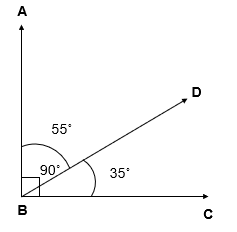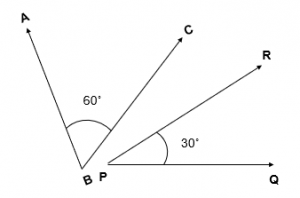# What is Complementary Angles – Complementary Angles Examples

What is Complementary Angles?

Two Angles are said to be Complementary Angles, if the sum of their measure is 90˚.

### Complementary Angles Examples:

• In this figure, two angles i.e, 55˚ and 35˚ are Complementary Angles.
As, 55˚ + 35˚ = 90˚• In this figure, two angles i.e, 60˚ and 30˚ are Complementary Angles.
As, 60˚ + 30˚ = 90˚Example 1

If one of the two Complementary Angles is 24˚ , what is the measure of the other Angle ?

Explanation

Two Angles are said to be Complementary Angles, if the sum of their measure is 90˚.
Measure of one Angle = 24 ˚
Let the Measure of the Second Angle be =x ˚
Sum of Complementary Angles =90 ˚
In other words, 24˚ + x˚ = 90˚
x˚ = 90˚ – 24˚
x˚ = 66˚
Hence, the measure of the other Angle is = 66˚

Example 2

The difference in the measure of two Complementary Angles is 6˚ . Find the measure of Angles?

Explanation

Two Angles are said to be Complementary Angles, if the sum of their measure is 90˚.
Let the measure of one Complementary Angle = ( x )˚
Then, the measure of other Angle = ( x – 6 )˚
Sum of Complementary Angles = 90˚
x˚ + x˚ – 6˚ = 90˚
2x˚ = 90˚ + 6˚
2x˚ = 96˚
x = 96/2

x = 48
Hence, the measure of one Angle = ( x )˚ = 48˚
The measure of other Angle = ( x – 6 )˚ = ( 48 – 6)˚ = 42˚

Example 3

If two Complementary Angles are in the ratio 1 : 4  , find the measure of Angles?

Explanation

Two Angles are said to be Complementary Angles, if the sum of their measure is 90˚.
Let the measures of two Angles be ( 1a )˚ and ( 4a )˚
Sum of Complementary Angles = 90˚
( 1a )˚ + (4a )˚ = 90˚
( 5a )˚ = 90˚
a = 90/5

a = 18
Hence, measure of two Angles are:
( 1a )˚ = ( 1 x 18 )˚ = 18˚
( 4a )˚ = ( 4 x 18 )˚ = 72˚